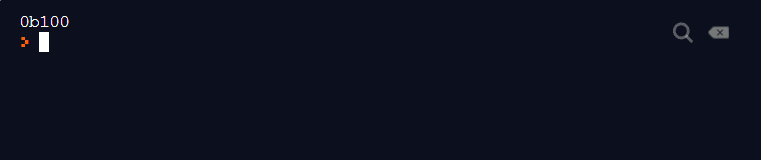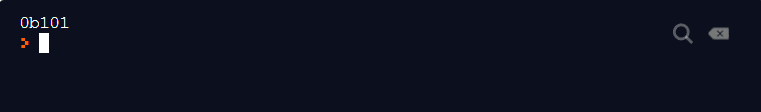We have learned about binary operators, unary operators, arithmetic, and string operators. This is how we do most of the operations, without worrying about the underlying machine level logic. Subsequently, in this tutorial, we will learn about Python Bitwise Operators and understand how the operations can happen with just binary numbers. Consequently, we will go through the following topics:-

• What is a Bitwise Operator in Python?
• Importance of Bitwise Operators
• Different Python Bitwise Operators
• Practice for Python Bitwise Operators

## What is a Bitwise Operator in Python?

Bitwise Operators are used to performing operations on binary patterns (1s and 0s ). When you perform an integer operation 2 + 3 on the screen, the computer will read it in binary form - 2 is represented as 10, and 3 is represented as 11 in binary format. Consequently, your calculation will look like -

10 + 11 = 101

Bitwise operators may look intimidating at first, as they convert everything to bits and we are not used to 1s and 0s. However, once you have understood them, they are very easy to work upon. Subsequently, let's see an example

3 x 2 = 6  If you perform the same operation in binary format -

011 x 10 = 110

If you notice, when we multiply something by 2, we simply do one left shift. Therefore, 011 will become 110.

Similarly, 0010 will become 0100. Now that you know this, can you see how easy it is to multiply by 2? Any guesses what will happen if we divide by 2? Well, you guessed it right, instead of the left shift, we will have the right shift. Consequently, the operators that make these operations on bits possible are known as bitwise operators. Subsequently, we will learn these left and right shifts in detail in the latter part of this tutorial.

Before we move ahead, let's find out why bitwise operators are essential. Additionally, we will also understand why we need to learn them.

### Importance of Bitwise operators

By now you would be wondering about the need to do bitwise operations. The arithmetic operation was working pretty well so far. A few of the reasons that will urge you to use the bitwise operators are:

• Execution Speed: As we have learned so far, the computer (compiler to be precise),  changes everything to 1s and 0s. So, why not take that load off the system?  As we have got advanced processors now, you will not see this difference in usual operations. However, if you are working on Embedded Systems programs, this becomes important.
• Manipulating Bits: A lot of the time, you would need to manipulate certain bits while working on embedded systems.
• For example, in sequence 111011, if you want to know the bit which has 0. You can do that using the bitwise operator (we will cover that later on how to do that).
• Error checking: Bitwise operators are used extensively in error checking. If you are sending out some bits to another computer on another server, it is prone to some errors. Subsequently, you can identify them using bitwise operators. This is important for embedded systems, and python is one of the prominent options for that.

By now, you would have got a good understanding of why we need bitwise operators. Subsequently, let's move ahead with types of bitwise operators.

### Different Python Bitwise Operators

Python offers several useful operators for performing bitwise operations. Subsequently, let's understand these in detail.

#### AND Bitwise Operators in Python

The & (AND ) operator is used to perform an AND between two bits. Additionally, a simple rule for AND is, if both the bits in consideration are 1, the answer is 1 else 0.

1 & 1 = 1

1 & 0 = 0

0 & 0 = 0

0 & 1 = 0

Now on the same lines, can you try 11 & 01? I hope you got the answer as 01.

Moreover, we discussed earlier, how to find which bit has got a 0. This is done using the AND operation.

• If you AND a number with 11111111 (considering 1 byte for now), if the resulting bit is 0, the error happens at that position.

In the following calculation, we are operating 100 and 101 using & bitwise operator in Python. You already know that to represent a binary number, we prefix it with 0b.

Therefore, you can directly write the following statement in the editor (native or cloud ) and run to get the output. If you are comfortable with the native IDE then you can download PyCharm or else, you can use cloud-based editor Repl also. Subsequently, in this post, we will be using Rep.it

Execute the following code in the editor:

print(0b100 & 0b101)Note: 100 is 4 in binary while 101 is 5. Additionally, the prefix 0b denotes that we are referring to the binary values and not the decimal 100 and 101.

As expected, we get the result as 4. You must notice that even though we executed the calculation in binary, we still got the result in decimal. Additionally, this is the default behavior in programming languages. Additionally, without explicitly referencing keywords, the results would be in decimal.

Just as we referred 0b to denote binary in input, to get the binary output, we need to mention the "bin " keyword.

Subsequently, execute the following to get the same result in binary:

print(bin(0b100 & 0b101))

This will bring out the following result:More examples:

• 12 & 14 = 1100 & 1110 = 1100 = 12
• 23 & 15 = 10111 & 01111 = 00111 = 7
• 10 & 12 = 1010 & 1100 = 1000 = 8

#### OR Bitwise Operators in Python

The | (OR ) operator is used to perform the OR operation on two bits. Moreover, a simple rule for OR is, the resultant bit is 0 if and only if both the bits in consideration are 0 else the result is 1.

1 | 1 = 1

1 | 0 = 1

0 | 1 = 1

0 | 0 = 0

In the below example, we are operating number 5 (101 ) with 4 (100 ) and expect the answer to be 101 (5 ) that is only the middle bit with 0.As expected, the answer to 5 | 4 is 5.

More examples:

• 12 | 14 = 1100 | 1110 = 1110 = 14
• 23 | 15 = 10111 | 01111 = 11111 = 31
• 10 | 12 = 1010 | 1100 = 1110 =  14

#### Not Python Bitwise Operator

The ~ (NOT ) operator is a very simple operator and works just as its name suggests. Additionally, it flips the bit from 0 to 1 and from 1 to 0. But when used in programming like Python, this operator is used for returning the complement of the number.

• Therefore, ~10 = -11 and not 01. Moreover, prior knowledge of 1's complement and 2's complement is necessary to understand the role of this operator here.
• To understand the concept, you should know that python returns the 2's complement of the number. 2's complement of the number is ~(num + 1).
• Therefore ~10 is returned as ~(10 + 1). That's all you need to know. Just remember the shortcut ~(num + 1)

Execute the following code in the editor to verify the output for not operation of 24 (11000 )

print(not(0b11000))The answer comes to -25 as expected.

More examples:

• ~13  (0b01101) =  -14
• ~16 (0b010000) =  -17
• ~31 (0b011111) = -32

#### XOR Python Bitwise Operator

The ^ (XOR ) operator is used to XOR two bits that are under operation. XOR operation is very simple. If two bits are the same then the resulting bit is 0 else 1.

1 ^ 1 = 0

1 ^ 0 = 1

0 ^ 1 = 1

0 ^ 0 = 0

So 1010 ^ 1011 will result in 0001.

The same is demonstrated below by executing the following lines:

print(0b1010 ^ 0b1011)XOR operator is extremely useful in computer science and embedded systems field. It is used very extensively in logical calculations to make the process faster. For example, as you saw above, 10 and 11 produce a result of 1 when XOR is done on them. Therefore, if I want to find out if two numbers are consecutive or not, I can just XOR them and check whether the result is 1 or not.

More examples:

• 12 ^ 14 = 1100 ^ 1110 = 0010 = 2
• 23 ^ 15 = 10111 ^ 01111 = 11000 = 24
• 10 ^ 12 = 1010 ^ 1100 = 0110 =  6

#### Left Shift in Python

The << (Bitwise left shift ) operator, as its name suggests, shifts the bits towards the left to a number represented to the right side of this operator.

For example, 1 << 2 will shift 1 towards left for 2 values. In bit terms, it will be presented as follows:

• 1 = 0001.
• 1 << 2: 0001 << 2 = 0100 i.e. 4.

Execute the below-given code to see the same in the result:

print(0b0001 << 2)As expected the answer to 1 << 2 is 4.

More examples:

• 14 << 1 = 01110 << 1 = 11100 = 28
• 24 << 4 = 000011000 << 4 = 110000000 = 384
• 19 << 3 = 00010011 << 3 =  10011000 = 152

By now you will have figured out that when you represent the binary numbers in an editor, you will need to prefix 0b before you execute above code.

#### Right Shift in Python

The >> (right-shift ) operator, as its name suggests, shift the bits towards the right to a number represented to the right side of the operator.

For example, 10 >> 2 will shift the bits (1010) towards the right by 2.

• 10 = 1010
• 10 >> 2: 1010 >> 2 = 0010 = 2

Let's confirm the same by executing the following lines:

print(0b1010 >> 2)As expected, the answer to 10 >> 2 is 2.

More examples:

• 14 >> 1 = 01110 >> 1 = 00111 = 7
• 24 >> 4 = 000011000 >> 4 = 00001 = 1
• 19 >> 3 = 00010011 >> 3 =  000010 = 2

This brings us to the end of learning bitwise operators in Python or any programming language for that matter. The key to bitwise operators is just not knowing their definitions but to be able to implement them in your programs. To be efficient in bitwise operators, practice a lot using bit manipulations, and explore how they behave in different cases. Once you get a hold of these operators, they are the best and fastest way to solve a problem.

## Practice:

For your practice, I have mentioned a few operations using the operators discussed in this post. These operands have not been written into bits as exploring them will help you grip over the concept.

Perform the following operations and analyze, explore, and observe the results.

• 35 & 27
• -23 | 23
• 18 >> 5
• -43 << 3
• 17 ^ 42

## Key Takeaways:

• Bitwise operators are the operators that work on the bit level in a programming language such as Python.
• Additionally, Bitwise operators are used very widely in embedded systems, networking infrastructures, and programming.
• Moreover, Bitwise operators provide faster, space-efficient, and error checking methods.
• The bitwise operators used in python are:
• The AND operator.
• The OR operator.
• The NOT operator.
• The XOR operator.
• The Left Shift operator.
• The Right Shift operator.ReviewersLakshay Sharma